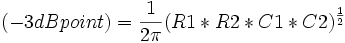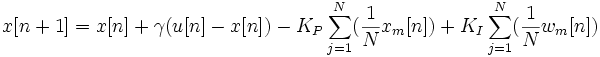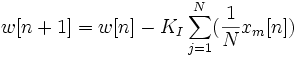# The sandbox

(Difference between revisions)

## test$(-3dB point) = \frac{1}{2\pi}(R1*R2*C1*C2)^\frac{1}{2}$$x[n+1] = x[n] + \gamma(u[n]-x[n]) - K_P\sum_{j=1}^N(\frac{1}{N}x_m[n]) + K_I\sum_{j=1}^N(\frac{1}{N}w_m[n])$$w[n+1] = w[n] - K_I\sum_{j=1}^N(\frac{1}{N}x_m[n])$